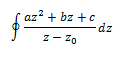# Polar Coordinates to evaluate integrals

## Homework Statement

Use Polar coordinates to evaluatewere C denotes the unit circle about a fixed point Z0 in the complex plane

## The Attempt at a Solution

I've only used polar integrals to convert an integral in sin and cos into one in therms of z, find the residues and then use the residue theorum to evaluate the integral so I am not really sure where to go with this question? Any help would be greatly appreciated!

## Answers and Replies

HallsofIvy
Science Advisor
Homework Helper
Let $z= z_0+ e^{i\theta}$.

Have I done it correctly if I end up with a final answer of
2∏i(aZ02 + bZ0 + c)
Thanks!

HallsofIvy
Science Advisor
Homework Helper
Yes, in fact there is the "Cauchy integral formula" that says
$$\oint \frac{f(z)}{z-z_0} dz= 2\pi if(z_0)$$

Perhaps this problem was intended as an introduction to that.

Ah yes we have done that previously, I think I just need to do practise questions to bring all the theory together. Cheers